# LQRSP – Sparsity-Promoting Linear Quadratic Regulator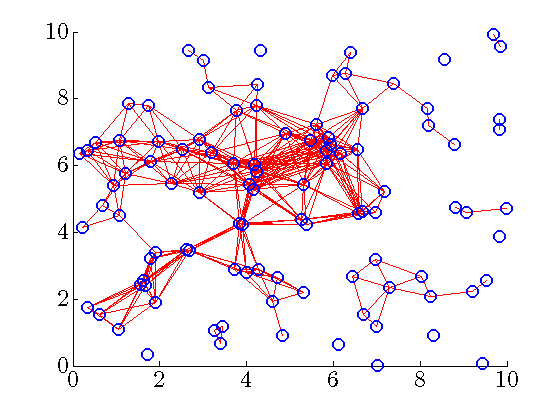January 2012 Matlab Files Download all Matlab files Presentation 2012 MIT LIDS Talk

## Introduction

lqrsp.m is a Matlab function for the design of sparse and block sparse state-feedback gains that minimize the variance amplification (i.e., the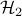norm) of distributed systems. Our long-term objective is to develop a toolbox for sparse feedback synthesis. This will allow users to identify control configurations that strike a balance between the performance and the sparsity of the controller and to examine the influence of different control configurations on the performance of distributed systems.

The design procedure consists of two steps:

1. Identification of sparsity patterns by incorporating sparsity-promoting penalty functions into the optimal control problem.

2. Optimization of feedback gains subject to structural constraints determined by the identified sparsity patterns.

## Problem formulation

Consider the following control problemwhere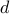is the exogenous input signal and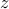is the performance output. The matrix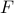is a state feedback gain,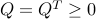and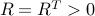are the state and control performance weights, and the closed-loop system is given by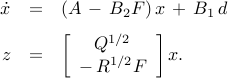We study the sparsity-promoting optimal control problem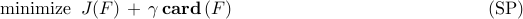in which the closed-loopnorm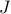is augmented by a function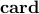that counts the number of nonzero elements in the feedback gain matrix. For example,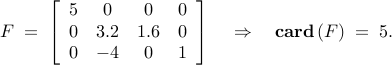As the parameter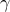varies over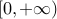, the solution traces the optimal trade-off path between the quadratic performance and the feedback gain sparsity.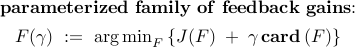Increased emphasis on sparsity encourages sparser control architectures at the expense of deteriorating system's performance. For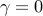the optimal controller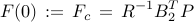is obtained from the positive definite solution of the algebraic Riccati equation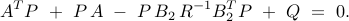Control architectures for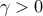depend on interconnections in the distributed plant and the state and control performance weights.

After a desired level of sparsity is achieved, we fix the sparsity structure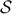and find the optimal structured feedback gain.## Relaxations of cardinality function

In addition to the cardinality function, we also consider several sparsity-promoting relaxations including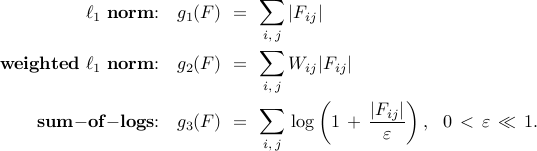To illustrate the sparsity-promoting properties of these relaxations, let us consider the problem of finding the sparsest feedback gain that provides a given level of performance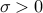. Convex (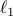and weightednorm) and non-convex (sum-of-logs) relaxations of the cardinality function lead to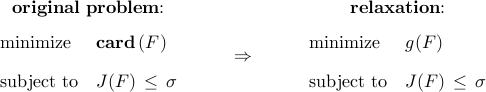The solution of the relaxed problem is the intersection of the constraint set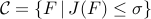and the smallest sub-level set of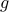that touches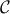.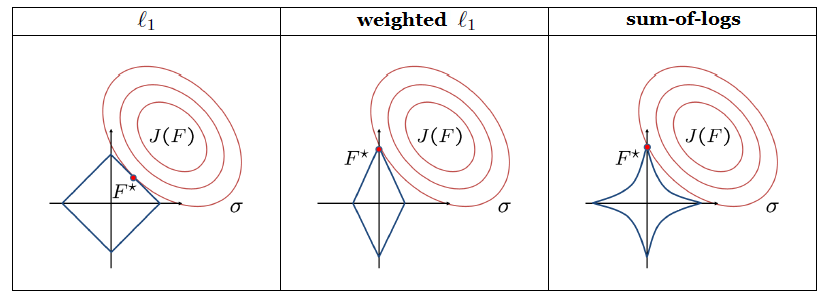## Alternating direction method of multipliers (ADMM)

ADMM is a simple but powerful algorithm well-suited to large optimization problems. It blends the separability of the dual decomposition with the superior convergence of the method of multipliers. In the sparsity-promoting linear quadratic regulator problem, we use ADMM as the general purpose algorithm that

1. identifies sparsity patterns

2. provides good initial condition for structured design.

The algorithm consists of four steps:

• Step 1: introduce additional variable/constraint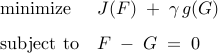• Step 2: introduce the augmented Lagrangian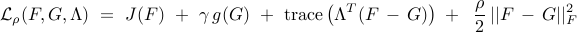• Step 3: use ADMM for the augmented Lagrangian minimization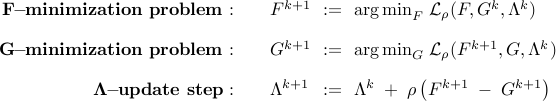• Step 4: polishing - solve the structuredproblem for the identified sparsity pattern.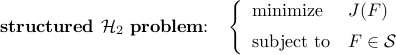## Sketch of the algorithm

### Solution to the G-minimization problem

Since all of the above mentioned sparsity-promoting penalty functions can be written as a summation of componentwise functions, we can decompose the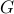-minimization problem into sub-problems expressed in terms of the individual elements of,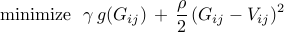where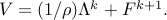This separability of the-minimization problem facilitates development of element-wise analytical solutions.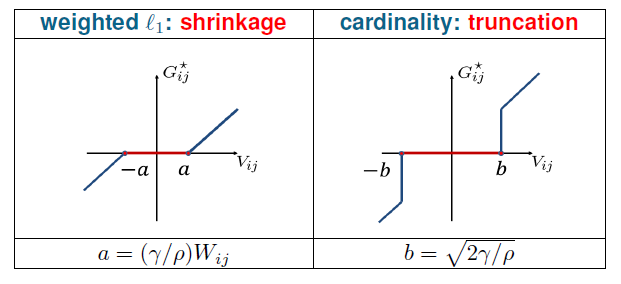Left panel: solution of the-minimization sub-problem for weighted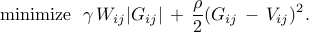Right panel: solution of the-minimization sub-problem for cardinality function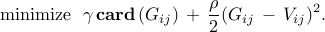For sum-of-logs function, the solution to the-minimization sub-problem depends on the parameters,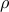,. For example, the solution of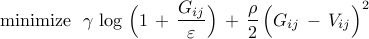with,resembles the shrinkage operator for small values of(e.g.,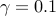), and it resembles the truncation operator for large values of(e.g.,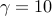).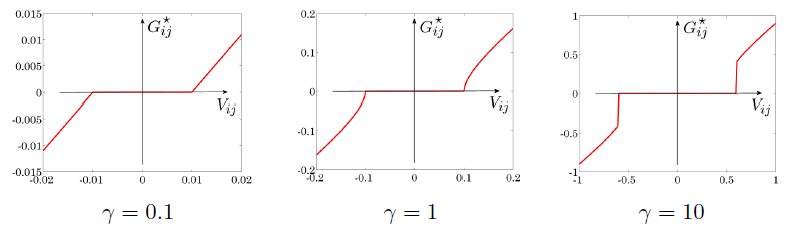### Solution to the F-minimization problem

The necessary conditions for the optimality of the-minimization problem are given by the three coupled nonlinear equations in the unknowns matrices,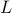, and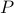,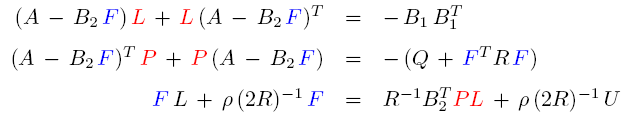For a fixed, the first two equations are Lyapunov equations for the closed-loop controllability and observability gramiansand, respectively; for fixedand, the third equation is a Sylvester equation for. This structure of the necessary conditions for the optimality motivates the following iterative scheme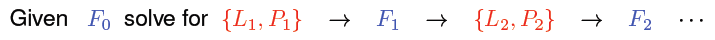We have shown that the difference between the feedback gains in two consecutive steps provides a descent direction. This observation in conjunction with standard line search has allowed us to establish convergence of the iterative scheme.

### Polishing

The necessary conditions for the optimality of the structured linear quadratic regulator problem are given by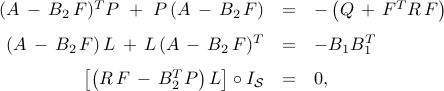wheredenotes the entry-wise multiplication of two matrices, and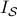is the structural identity. For example,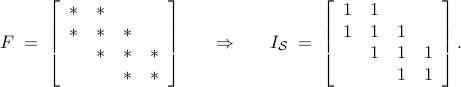We have used Newton's method and employed the conjugate gradient scheme to compute the Newton direction efficiently.

## Extension: Block sparsity

In many problems it is of interest to design feedback gains that have block sparse structure. In view of this, we have also considered the optimal control problem that promotes sparsity of the feedback gain at the level of the submatrices instead of at the level of the individual elements.

The function that counts the number of nonzero submatrices is given by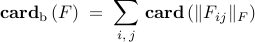where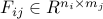and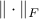is the Frobenius norm. We have also examined the following relaxations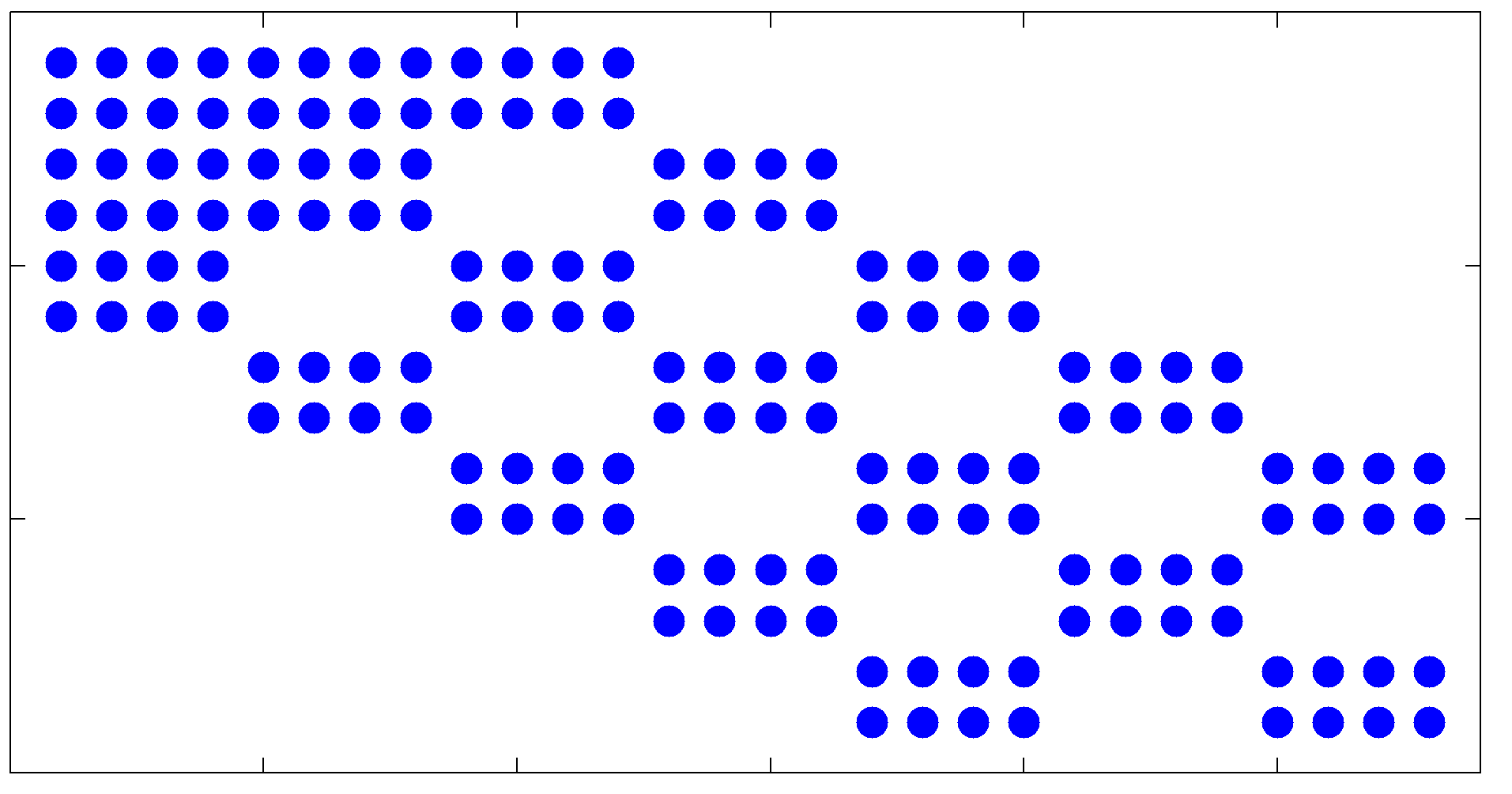An example of a block sparse feedback gain with submatrices of size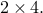Additional information is provided in the block sparsity example.

## Description of lqrsp.m

Matlab Syntax
```>> solpath = lqrsp(A,B1,B2,Q,R,options);
```

Our Matlab function lqrsp.m takes the matrices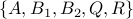and the input options and returns the-parameterized family of solutions to both the sparsity-promoting optimal control problem (SP) and the structuredproblem (SH2). Input options allows users to specify the following parameters

1. sparsity-promoting penalty function:,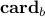, or one of the relaxations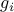with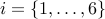;

2. values ofand;

3. maximum number of ADMM iterations;

4. maximum number of re-weighted iterations (if weightedor weighted sum of Frobenius norms is used);

5. block size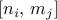of the submatrix(if block sparsity is desired).

The-parameterized output solpath is a structure that contains

1. solpath.F – feedback gains resulting from the control problem (SP);

2. solpath.Fopt – feedback gains resulting from the structured control problem (SH2);

3. solpath.J – quadratic performance of the solutions to (SP);

4. solpath.Jopt – optimal quadratic performance of the solutions to (SH2);

5. solpath.nnz – number of nonzero elements (blocks) of optimal sparse (block sparse) feedback gains;

6. solpath.gam – values of sparsity-promoting parameter.

```help lqrsp
```
```  Sparsity-Promoting Linear Quadratic Regulator

Written by Fu Lin, January 2012

Description:

The sparsity-promoting linear quadratic regulator problem is solved using
the alternating direction method of multipliers (ADMM)

minimize J(F) + gamma * g(F)             (SP)

where J is the closed-loop H2 norm, g is a sparsity-promoting penalty
function, and gamma is the parameter that emphasizes the importance of
sparsity.

After the sparsity pattern has been identified, the structured H2 problem
is solved

minimize    J(F)
(SH2)
subject to  F belongs to identified sparsity pattern

Syntax:

solpath = lqrsp(A,B1,B2,Q,R,options)

Inputs:

(I)  State-space representation matrices {A,B1,B2,Q,R};

(II) Options

a) options.method specifies the sparsity-promoting penalty function g

options.method = 'card'     --> cardinality function,
= 'l1'       --> l1 norm,
= 'wl1'      --> weighted l1 norm,
= 'slog'     --> sum-of-logs function,
= 'blkcard'  --> block cardinality function,
= 'blkl1'    --> sum of Frobenius norms,
= 'blkwl1'   --> weighted sum of Frobenius norms,
= 'blkslog'  --> block sum-of-logs function;

b) options.gamval         --> range of gamma values;
c) options.rho            --> augmented Lagrangian parameter;
d) options.maxiter        --> maximum number of ADMM iterations;
e) options.blksize        --> size of block sub-matrices of F;
f) options.reweightedIter --> number of iterations for the reweighted
update scheme.

The default values of these fields are

options.method         = 'wl1';
options.gamval         = logspace(-4,1,50);
options.rho            = 100;
options.maxiter        = 1000;
options.blksize        = [1 1];
options.reweightedIter = 3.

Output:

solpath -- the solution path parameterized by gamma -- is a structure
that contains:

(1) solpath.F    --> feedback gains resulting from the control problem
(SP);
(2) solpath.Fopt --> feedback gains resulting from the structured control
problem (SH2);
(3) solpath.J    --> quadratic performance of the solutions to (SP);
(4) solpath.Jopt --> optimal quadratic performance of the solutions to
(SH2);
(5) solpath.nnz  --> number of nonzero elements (blocks) of optimal
sparse (block sparse) feedback gains;
(6) solpath.gam  --> values of sparsity-promoting parameter gamma.

Reference:

F. Lin, M. Fardad, and M. R. Jovanovic
``Design of optimal sparse feedback gains via the alternating direction method of multipliers''
IEEE Trans. Automat. Control, vol. 58, no. 9, pp. 2426-2431, September 2013.

http://www.umn.edu/~mihailo/software/lqrsp/

```

## Acknowledgements

We would like to thank Stephen Boyd for encouraging us to develop this software.

This project is supported in part by the National Science Foundation under

• CAREER Award CMMI-0644793

• Award CMMI-0927509

• Award CMMI-0927720.